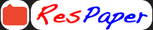Trending ▼   ResFinder# CBSE Class 10 Pre Board 2021 : Mathematics (Guru Teg Bahadur Public School (GTBPS), Durgapur)

8 pages, 62 questions, 0 questions with responses, 0 total responses,30Ayan Ahmed Bluebells School International, Kailash Colony, New Delhi
+Fave Message
 Home > ayanahmed >   F Also featured on: School Page cbse10_pre_boards and 1 moreFormatting page ...

GURU TEG BAHADUR PUBLIC SCHOOL DURGAPUR PRE-BOARD EXAMINTION: 2020 21 CLASS: X TIME: 3 HR SUBJECT : MATHEMATICS(Standard) FM: 80 General Instructions: 1. This question paper contains two part A and B. 2. Both part A and part B have internal choices. Part A: 1. It consists of two sections I and II. 2. Section I has 16 question of 1 mark each. Internal choice is provided in 5 questions. 3. Section II has 4 questions on case study. Each case study has 5 case-based sub-parts. An examinee is to attempt any 4 out of 5 sub-parts. Part B: 1. Question No. 21 to 26 are very short answer type questions of 2 mark each. 2. Question No.27 to 33 are short answer type questions of 3 marks each. 3. Question No34 to 36 are long answer type questions of 5 marks each. 4. Internal choice is provided in 2 questions of 2 marks ,2 questions of 3 marks and 1 question of 5 marks. Part A Section-1 Section 1 has 16 questions of 1 mark each. Internal choice is provided in 5 questions. 1. After how many places decimal expansion of the rational number will terminate? OR Write the decimal expansion of 2. If the sum of the zeros of the quadratic polynomial kx2 + 2x+3k is equal to the product of its zeros then find the value of k. 3. Find the value of k for which the following pair of linear equations have infinitely many solutions: 2x+3y = 7 , (k 1)x + (k+2)y = 3k. 4. The difference between two numbers is 26 and one number is three times the other. Find the numbers. 1Formatting page ...Formatting page ...Formatting page ...Formatting page ...Formatting page ...Formatting page ...Formatting page ...Additional Info : CBSE Class 10 Exam 2020 : Mathematics
Tags : CBSE Class 10 Exam 2020 : Mathematics,